# Nonlinear Optical Effects

The response of any dielectric to light becomes nonlinear for intense electromagnetic fields, and optical fibers are no exception. Even though silica is intrinsically not a highly nonlinear material, the waveguide geometry that confines light to a small cross section over long fiber lengths makes nonlinear effects quite important in the design of modern lightwave systems. We discuss in this tutorial the nonlinear phenomena that are most relevant for fiber-optic communications.

#### 1. Stimulated Light Scattering

Rayleigh scattering, discussed in the optical fiber loss tutorial, is an example of elastic scattering for which the frequency (or the photon energy) of scattered light remains unchanged. By contrast, the frequency of scattered light is shifted downward during inelastic scattering. Two examples of inelastic scattering are Raman scattering and Brillouin scattering. Both of them can be understood as scattering of a photon to a lower energy photon such that the energy difference appears in the form of a phonon. The main difference between the two is that optical phonons participate in Raman scattering, whereas acoustic phonons participate in Brillouin scattering. Both scattering processes result in a loss of power at the incident frequency. However, their scattering cross sections are sufficiently small that loss is negligible at low power levels.

At high power levels, the nonlinear phenomena of stimulated Raman scattering (SRS) and stimulated Brillouin scattering (SBS) become important. The intensity of the scattered light in both cases grows exponentially once the incident power exceeds a threshold value. SRS and SBS were first observed in optical fibers during the 1970s. Even though SRS and SBS are quite similar in their origin, different dispersion relations for acoustic and optical phonons lead to the following differences between the two in single-mode fibers: (i) SBS occurs only in the backward direction whereas SRS can occur in both directions; (ii) The scattered light is shifted in frequency by about 10 GHz for SBS but by 13 THz for SRS (this shift is called the Stokes shift); and (iii) the Brillouin gain spectrum is extremely narrow (bandwidth < 100 MHz) compared with the Raman-gain spectrum that extends over 20-30 THz. The origin of these differences lies in a relatively small value of the ratio vA/c (~ 10-5), where vA is the acoustic velocity in silica and c is the velocity of light.

Stimulated Brillouin Scattering

The physical process behind Brillouin scattering is the tendency of materials to become compressed in the presence of an electric field - a phenomenon termed electrostriction. For an oscillating electric field at the pump frequency Ωp, this process generates an acoustic wave at some frequency Ω. Spontaneous Brillouin scattering can be viewed as scattering of the pump wave from this acoustic wave, resulting in creation of a new wave at the pump frequency Ωs. The scattering process must conserve both the energy and the momentum. The energy conservation requires that the Stokes shift Ω equals ωpωs. The momentum conservation requires that the wave vectors satisfy kA = kp - ks. Using the dispersion relation |kA| = Ω/vA, where vA is the acoustic velocity, this condition determines the acoustic frequency as

Ω =  |kA|vA = 2vA|kp| sin(θ/2)

where  |kp≈ |ks| was used and θ represents the angle between the pump and scattered waves. Note that Ω vanishes in the forward direction (θ = 0) and is maximum in the backward direction (θ = π). In single-mode fibers, light can travel only in the forward and backward directions. As a result, SBS occurs in the backward direction with a frequency shift ΩB = 2vA|kp|. Using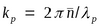, where λp is the pump wavelength, the Brillouin shift is given by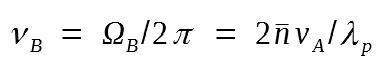where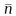is the mode index. Using vA = 5.96 km/s and= 1.45 as typical values for silica fibers, νB = 11.1 GHz at λp = 1.55 μm. This equation shows that νB scales inversely with the pump wavelength.

Once the scattered wave is generated spontaneously, it beats with the pump and creates a frequency component at the beat frequency ωp - ωs, which is automatically equal to the acoustic frequency Ω. As a result, the beating term acts as source that increases the amplitude of the sound wave, which in turn increases the amplitude of the scattered wave, resulting in a positive feedback loop. SBS has its origin in this positive feedback, which ultimately can transfer all power from the pump to the scattered wave. The feedback process is governed by the following set of two coupled equations: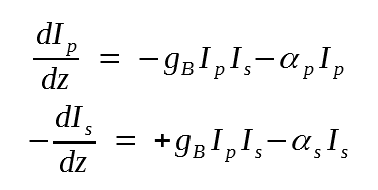where Ip and Is are the intensities of the pump and Stokes fields, gB is the SBS gain, and αp and αs account for fiber losses.

The SBS gain gB is frequency dependent because of a finite damping time TB of acoustic waves (the lifetime of acoustic phonons). If the acoustic waves decay as exp(-t/TB), the Brillouin gain has a Lorentzian spectral profile given by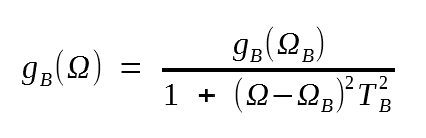The following figure shows the Brillouin gain spectra at λp = 1.525 μm for three different kinds of single-mode silica fibers.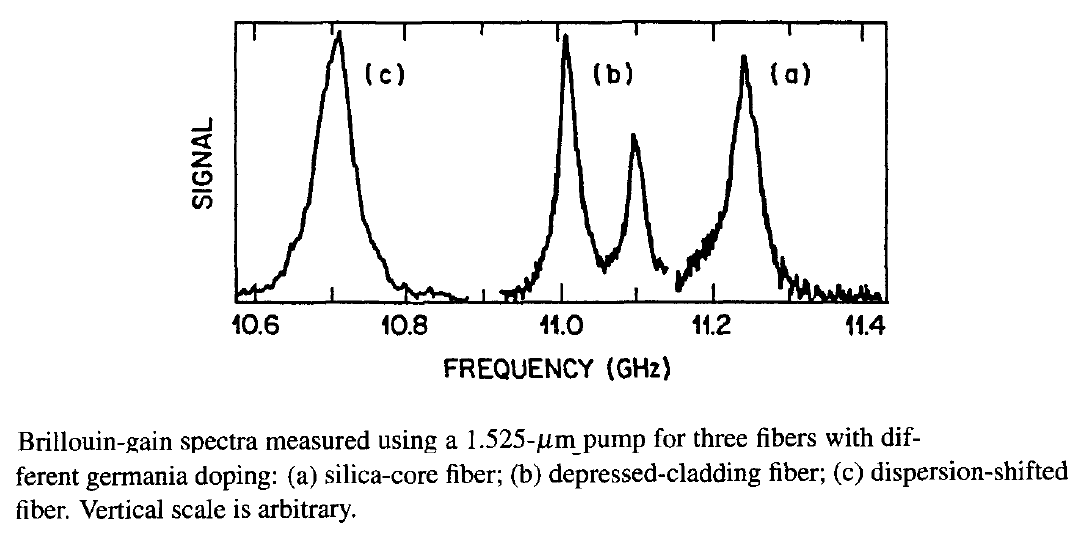Both the Brillouin shift νB and the gain bandwidth ΔνB can vary from fiber to fiber because of the guided nature of light and the presence of dopants in the fiber core. The fiber labeled (a) has a core of nearly pure silica (germania concentration of about 0.3% per mole). The measured Brillouin shift νB = 11.25 GHz is in agreement with its equation. The Brillouin shift is reduced for fibers (b) and (c) of a higher germania concentration in the fiber core. The double-peak structure for fiber (b) results from inhomogeneous distribution of germania within the core. The gain bandwidth in the figure is larger than that expected for bulk silica (ΔνB ≈ 17 MHz at λp = 1.525 μm). A part of the increase is due to the guided nature of acoustic modes in optical fibers. However, most of the increase in bandwidth can be attributed to variations in the core diameter along the fiber length.  Because such variations are specific to each fiber, the SBS gain bandwidth is generally different for different fibers and can exceed 100 MHz; typical values are ~ 50 MHz for λp near 1.55 μm.

The peak value of the Brillouin gain in the last equation occurs for Ω = ΩB and depends on various material parameters such as the density and the elasto-optic coefficient. For silica fibers gB ≈ 5 x 10-11 m/W. The threshold power level for SBS can be estimated by solving the equations and finding at what value of Ip and Is grows from noise to a significant level. The threshold power Pth = IpAeff , where Aeff is the effective mode area, satisfies the condition

gBPthLeff /Aeff ≈ 21

where Leff is the effective interaction length defined as

Leff  = [1 - exp(- αL)]/α

and α represents fiber losses. For optical communication systems  Leff can be approximated by 1/α as αL >> 1 in practice. Using Aeff = πw2, where w is the spot size, Pth can be as low as 1mW depending on the values of w and α. Once the power launched into an optical fiber exceeds the threshold level, most of the light is reflected backward through SBS. Clearly, SBS limits the launched power to a few milliwatts because of its low threshold.

The preceding estimate of Pth applies to a narrowband CW beam as it neglects the temporal and spectral characteristics of the incident light. In a lightwave system, the signal is in the form of a bit stream. For a single short pulse whose width is much smaller than the phonon lifetime, no SBS is expected to occur. However, for a high-speed bit stream, pulses come at such as fast rate that successive pulses build up the acoustic wave, similar to the case of a CW beam, although SBS threshold increases. The exact value of the average threshold power depends on the modulation format (RZ versus NRZ) and is typically ~5 mW. It can be increased to 10 mW or more by increasing the bandwidth of the optical carrier to >200 MHz through phase modulation. SBS does not produce interchannel crosstalk in WDM systems because the 10-GHz frequency shift is much smaller than typical channel spacing.

Stimulated Raman Scattering

Spontaneous Raman scattering occurs in optical fibers when a pump wave is scattered by the silica molecules. It can be understood using the energy-level diagram shown in (b) of the following figure.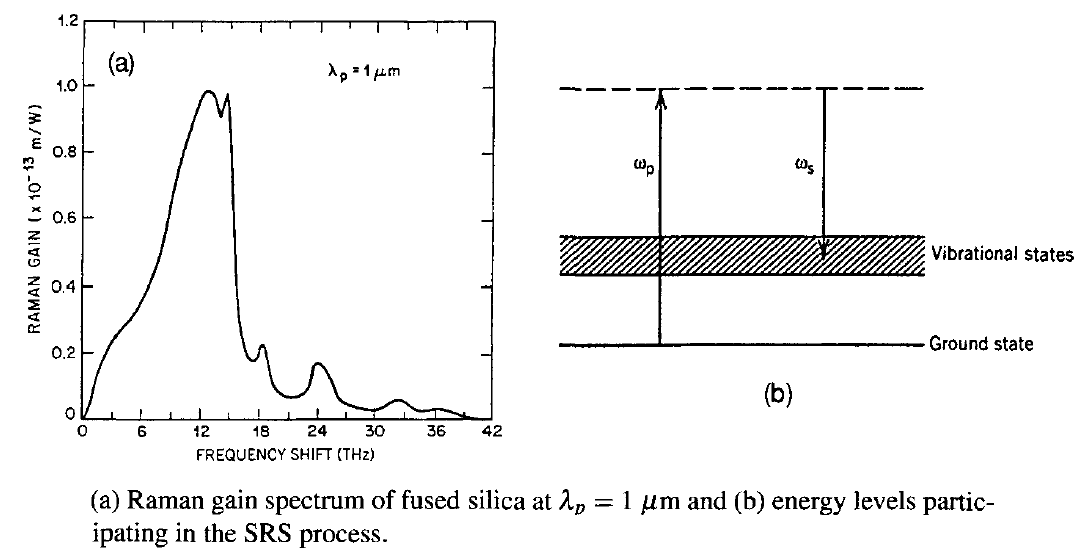Some pump photons give up their energy to create other photons of reduced energy at a lower frequency; the remaining energy is absorbed by silica molecules, which end up in an excited vibrational state. An important difference from Brillouin scattering is that the vibrational energy levels of silica dictate the value of the Raman shift ΩR = ωp - ωs. As an acoustic wave is not involved, spontaneous Raman scattering is an isotropic process and occurs in all directions.

Similar to the SBS case, the Raman scattering process becomes stimulated if the pump power exceeds a threshold value. SRS can occur in both the forward and backward directions in optical fibers. Physically speaking, the beating of the pump and with the scattered light in these two directions creates a frequency component at the beat frequency ωp - ωs, which acts as a source that derives molecular oscillations. Since the amplitude of the scattered wave increases in response to these oscillations, a positive feedback loop sets in. In the case of forward SRS, the feedback process is governed by the following set of two coupled equations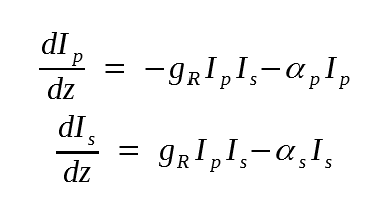where gR is the SRS gain. In the case of backward SRS, a minus sign is added in front of the derivative in the 2nd equation above, and this set of equations becomes identical to the SBS case.

The spectrum of the Raman gain depends on the decay time associated with the excited vibrational state. In the case of a molecular gas or liquid, the decay time is relatively long (~ 1 ns), resulting in a Raman-gain bandwidth of ~1 GHz. In the case for optical fibers, the bandwidth exceeds 10 THz. The figure above shows the Raman-gain spectrum of silica fibers. The broadband and multipeak nature of the spectrum is due to the amorphous nature of glass. More specifically, vibrational energy levels of silica molecules merge together to form a band. As a result, the Stokes frequency ωs can differ from the pump frequency ωp over a wide range. The maximum gain occurs when the Raman shift ΩR ≡ ωp - ωs is about 13 THz. Another major peak occurs near 15 THz while minor peaks persist for values of ΩR as large as 35 THz. The peak value of the Raman gain gR is about 1 x 10-13 m/W at a wavelength of 1 μm. This value scales linearly with ωp (or inversely with the pump wavelength λp), resulting in gR 6 x 10-13 m/W at 1.55 μm.

Similar to the case of SBS, the threshold power Pth is defined as the incident power at which half of the pump power is transferred to the Stokes field at the output end of a fiber of length L. It is estimated from

gRPthLeff /Aeff ≈ 16

where gR is the peak value of the Raman gain. As before, Leff can be approximated by 1/α. If we replace Aeff by πw2, where w is the spot size, Pth for SRS is given by

Pth ≈ 16α(πw2)/gR

If we use πw2 = 50 μm2 and α = 0.2 dB/km as the representative values, Pth is about 570 mW near 1.55 μm. It is important to emphasize that this equation provides an order-of-magnitude estimate only as many approximations are made in its derivation.

As channel powers in optical communication systems are typically below 10 mW, SRS is not a limiting factor for single-channel lightwave systems. However, it affects the performance of WDM systems considerably; this will be covered in another tutorial.

Both SRS and SBS can be used to advantage while designing optical communication systems because they can amplify an optical signal by transferring energy to it from a pump beam whose wavelength is suitably chosen. SRS is especially useful because of its extremely large bandwidth. Indeed, the Raman gain is used routinely for compensating fiber losses in modern lightwave systems.

#### 2. Nonlinear Phase Modulation

The refractive index of silica was assumed to be power independent in the discussion of fiber modes. In reality, all materials behave nonlinearly at high intensities and their refractive index increases with intensity. The physical origin of this effect lies in the anharmonic response of electrons to optical fields, resulting in a nonlinear susceptibility. To include nonlinear refraction, we modify the core and cladding indices of a silica fiber as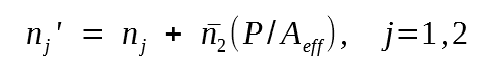whereis the nonlinear-index coefficient, P is the optical power, and Aeff is the effective mode area introduced earlier. The numerical value ofis about 2.6 x 10-20 m2/W for silica fibers and varies somewhat with dopants used inside the core. Because of this relatively small value, the nonlinear part of the refractive index is quite small (< 10-12  at a power level of 1 mW). Nevertheless, it affects modern lightwave systems considerably because of long fiber lengths. In particular, it leads to the phenomena of self- and cross-phase modulations.

Self-Phase Modulation

It we use first-order perturbation theory to see how fiber modes are affected by the nonlinear term in the equation above, we find that the mode shape does not change but the propagation constant becomes power dependent. It can be written as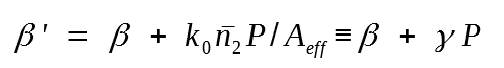whereis an important nonlinear parameter with values ranging from 1 to 5 W-1/km depending on the values of Aeff and the wavelength. Noting that the optical phase increases linearly with z, the γ term produces a nonlinear phase shift given bywhere P(z) = Pinexp(- αz) accounts for fiber losses and Leff is defined earlier.

In deriving this phase shift equation Pin was assumed to be constant. In practice, time dependence of Pin makes ΦNL to vary with time. In fact, the optical phase changes with time in exactly the same fashion as the optical signal. Since this nonlinear phase modulation is self-induced, the nonlinear phenomenon responsible for it is called self-phase modulation (SPM). SPM leads to frequency chirping of optical pulses. In contrast with the linear chirp, the frequency chirp is proportional to the derivative dPin/dt and depends on the pulse shape.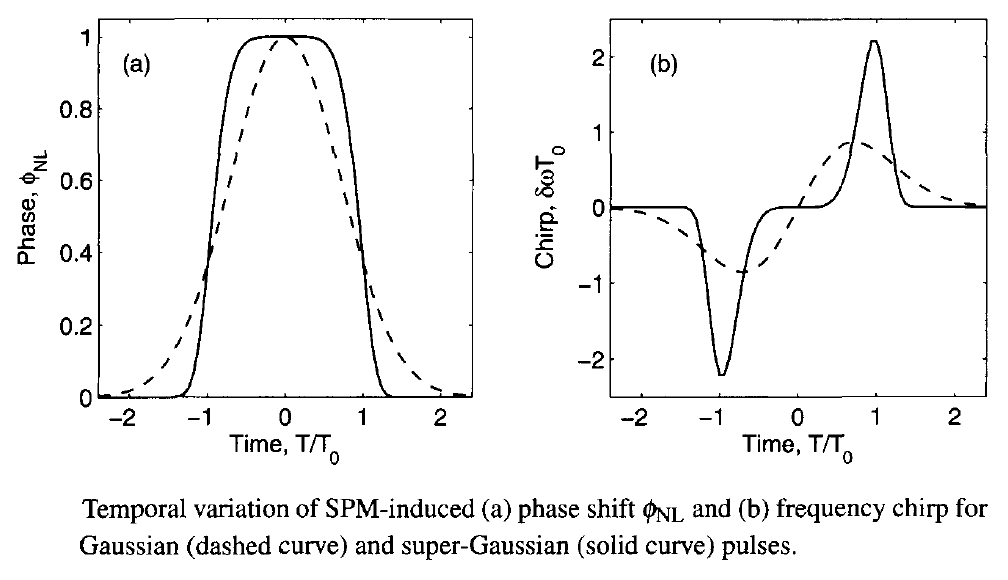The figure shows variation of (a) the nonlinear phase shift ΦNL and (b) the frequency chirp across the pulse for γPinLeff = 1 in the cases of a Gaussian pulse (m = 1) and a super-Gaussian pulse (m = 3). The SPM-induced chirp affects the pulse shape through GVD and often leads to additional pulse broadening. In general, spectral broadening of the pulse induced by SPM increases the signal bandwidth considerably and limits the performance of lightwave systems.

If fiber losses are compensated periodically using optical amplifiers, ΦNL should be multiplied by the number of amplifiers NA because the SPM-induced phase accumulates over multiple amplifiers. To reduce the impact of SPM in lightwave systems, it is necessary that ΦNL << 1. If we use ΦNL = 0.1 as the maximum tolerable value and replace Leff by 1/α for long fibers, this condition can be written as a limit on the input peak power as

Pin < 0.1α/(γNA)

For example, if γ = 2 W-1/km, NA = 10, and α = 0.2 dB/km, the input peak power is limited to below 2.2 mW. Clearly, SPM can be a major limiting factor for long-haul lightwave systems.

Cross-Phase Modulation

The intensity dependence of the refractive index can also lead to another nonlinear phenomenon known as cross-phase modulation (XPM). It occurs when two or more optical channels are transmitted simultaneously inside an optical fiber using the WDM technique. In such systems, the nonlinear phase shift for a specific channel depends not only on the power of that channel but also on the power of other channels. The phase shift for the jth channel becomes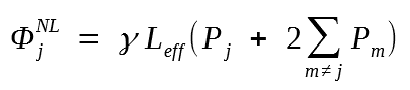where the sum extends over the number of channels. The factor of 2 in this equation has its origin in the form of the nonlinear susceptibility and indicates that XPM is twice as effective as SPM for the same amount of power. The total phase shift depends on the powers in all channels and would vary from bit to bit depending on the bit pattern of the neighboring channels. It we assume equal channel powers, the phase shift in the worst case in which all channels simultaneously carry 1 bits and all pulses overlap is given by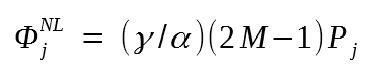It is difficult to estimate the impact of XPM on the performance of multichannel lightwave systems. The reason is that the preceding discussion has implicitly assumed that XPM acts in isolation without dispersive effects and is valid only for CW optical beams. In practice, pulses in different channels travel at different speeds. The XPM-induced phase shift can occur only when two pulses overlap in time. For widely separated channels they overlap for such a short time that XPM effects are virtually negligible. On the other hand, pulses in neighboring channels will overlap long enough for XPM effects to accumulate. These arguments show that the above equation cannot be used to estimate the limiting input power.

A common method for studying the impact of SPM and XPM uses a numerical approach. It is generalized to include the SPM and XPM effects by adding a nonlinear term. The resulting equation is known as the nonlinear Schrödinger equation and has the form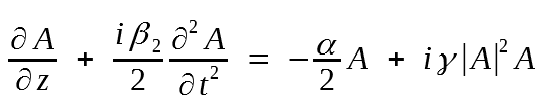where neglected the third-order dispersion and added the term containing α to account for fiber losses. This equation is quite useful for designing lightwave systems and will be used in later tutorials.

Since the nonlinear parameter γ depends inversely on the effective mode area, the impact of fiber nonlinearities can be reduced considerably by enlarging Aeff. Aeff is about 80 μm2 for standard fibers but reduces to 50 μm2 for dispersion-shifted fibers. A new kind of fiber known as large effective-area fiber (LEAF) has been developed for reducing the impact of fiber nonlinearities. The nonlinear effects are not always detrimental for lightwave systems. Numerical solutions of the equation above show that dispersion-induced broadening of optical pulses is considerably reduced in the case of anomalous dispersion. In fact, an optical pulse can propagate without distortion if the peak power of the pulse is chosen to correspond to a fundamental soliton.

#### 3. Four-Wave Mixing

The power dependence of the refractive index has its origin in the third-order nonlinear susceptibility denoted by Χ(3). The nonlinear phenomenon, known as four-wave mixing (FWM), also originates from Χ(3). If three optical fields with carrier frequencies ω1ω2, and ω3 copropagate inside the fiber simultaneously, Χ(3) generates a fourth field whose frequency  ω4 is related to other frequencies by a relation ω4 = ω1 ± ω2 ± ω3. Several frequencies  corresponding to different plus and minus sign combinations are possible in principle. In practice, most of these combinations do not build up because of a phase-matching requirement. Frequency combinations of the form ω4 = ω1 + ω2 - ω3 are often troublesome for multichannel communication systems since they can become nearly phase-matched when channel wavelengths lie close to the zero-dispersion wavelength. In fact, the degenerate FWM process for which ω1 = ω2 is often the dominant process and impacts the system performance most.

On a fundamental level, a FWM process can be viewed as a scattering proces in which two photons of energies ћω1 and ћω2 are destroyed, and their energy appears in the form of two new photons of energies ћω3 and ћω4. The phase-matching condition then stems from the requirement of momentum conservation. Since all four waves propagate in the same direction, the phase mismatch can be written as

Δβ(ω3) + β(ω4) - β(ω1) - β(ω2)

where β(ω) is the propagation constant for an optical field with frequency ω. In the degenerate case, ω2 = ω1ω3 = ω1 + Ω, and ω4 = ω1 - Ω, where Ω represents the channel spacing. Using the Taylor expansion, we find that the β0 and β1 terms cancel, and the phase mismatch is simply Δ = β2Ω2. The FWM process is completely phase matched when β2 = 0. When β2 is small (< 1 ps2/km) and channel spacing is also small (Ω < 100 GHz), this process can still occur and transfer power from each channel to its nearest neighbors. Such as power transfer not only results in the power loss for the channel but also induces interchannel crosstalk that degrades the system performance severely. Modern WDM systems avoid FWM by using the technique of dispersion management in which GVD is kept locally high in each fiber section even though it is low on average. Commercial dispersion shifted fibers are designed with a dispersion of about 4 ps/(km-nm), a value found large enough to suppress FWM.

FWM can also be useful for lightwave systems. It is often used for demultiplexing individual channels when time-division multiplexing is used in the optical domain. It can also be used for wavelength conversion. FWM in optical fibers is sometimes used for generating a spectrally inverted signal through the process of optical phase conjugation. This technique is useful for dispersion compensation.